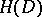# Fréchet space

(diff) ← Older revision | Latest revision (diff) | Newer revision → (diff)

A complete metrizable locally convex topological vector space. Banach spaces furnish examples of Fréchet spaces, but several important function spaces are Fréchet spaces without being Banach spaces. Among these are: the Schwartz spaceof all infinitely-differentiable complex-valued functions onthat decrease at infinity, as do all their derivatives, faster than any polynomial, with the topology given by the system of semi-normswhereandare non-negative integer vectors; the spaceof all holomorphic functions on some open subsetof the complex plane with the topology of uniform convergence on compact subsets of, etc.

A closed subspace of a Fréchet space is a Fréchet space; so is a quotient space of a Fréchet space by a closed subspace; a Fréchet space is a barrelled space, and therefore the Banach–Steinhaus theorem is true for mappings from a Fréchet space into a locally convex space. If a separable locally convex space is the image of a Fréchet space under an open mapping, then it is itself a Fréchet space. A one-to-one continuous linear mapping from a Fréchet space onto a Fréchet space is an isomorphism (an analogue of a theorem of Banach).

Fréchet spaces are so named in honour of M. Fréchet.

How to Cite This Entry:
Fréchet space. Encyclopedia of Mathematics. URL: http://encyclopediaofmath.org/index.php?title=Fr%C3%A9chet_space&oldid=14937
This article was adapted from an original article by V.M. Tikhomirov (originator), which appeared in Encyclopedia of Mathematics - ISBN 1402006098. See original article